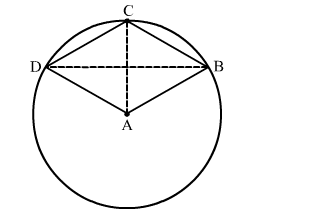# ABCD is a quadrilateral such that A is the centre of the circle passing through B, C and D.

Question:

$A B C D$ is a quadrilateral such that $A$ is the centre of the circle passing through $B, C$ and $D$. Prove that $\angle C B D+\angle C D B=\frac{1}{2} \angle B A D$.

Solution:

In the given figure, ABCD is a quadrilateral such that A is the centre of the circle passing through B, C and D.Join AC and BD.

We know that the angle subtended by an arc of a circle at the centre is double the angle subtended by it on the remaining part of the circle.

Here, arc CD subtends ∠CAD at the centre and ∠CBD at B on the circle.

Also, arc CB subtends ∠CAB at the centre and ∠CDB at D on the circle.

∴ ∠CAB = 2∠CDB           ...(2)

Adding (1) and (2), we get
CAD + CAB = 2(∠CBD + CDB)
⇒ BAD = 2(∠CBD + CDB)

$\Rightarrow \angle C B D+\angle C D B=\frac{1}{2} \angle B A D$

Hence, $\angle C B D+\angle C D B=\frac{1}{2} \angle B A D$.